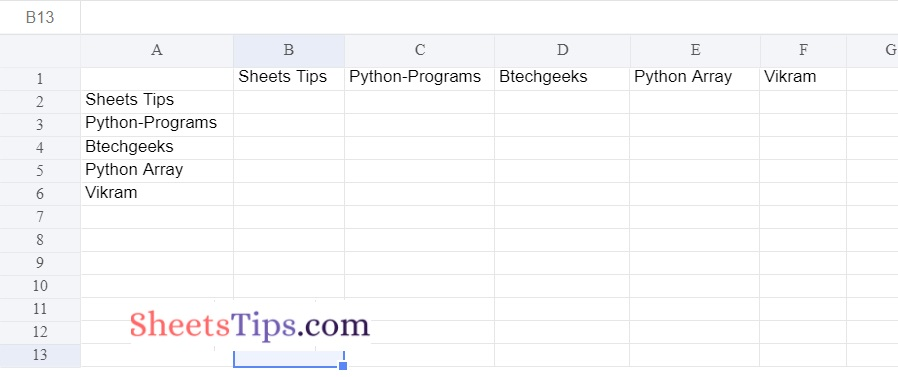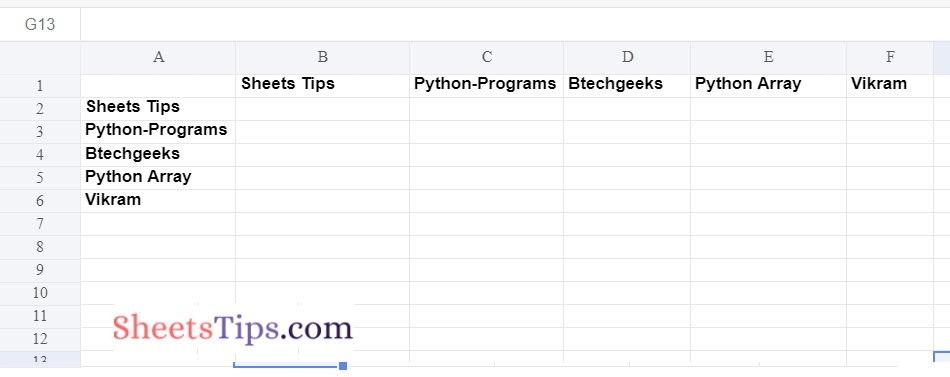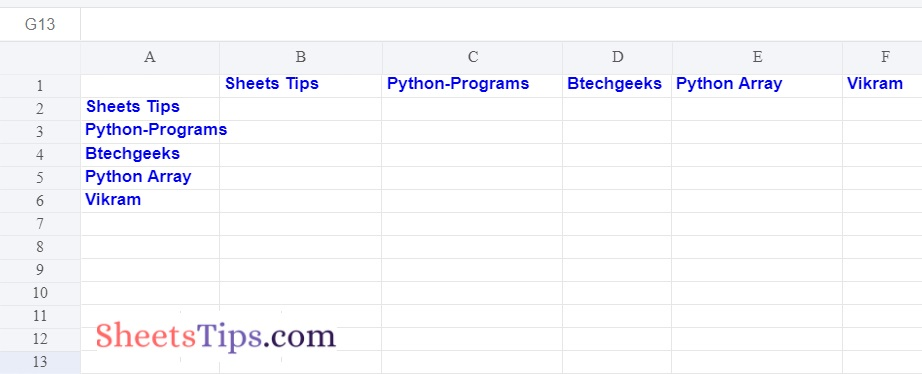# Python Program to Write to an Excel Sheet using xlwt module

In this article, we are going to write to an Excel Sheet using the xlwt module in Python

Xlwt Module:

Multiple spreadsheet operations can be performed with the xlwt module. Python, for example, can be used to write or modify data. In addition, the user may have to navigate through several sheets and get data based on some criteria, or edit some rows and columns, among other things.

## Program to Write to an Excel Sheet using xlwt module in Python

### Code #1: Writing an Excel Worksheet

Approach:

• Import the xlwt module using the import keyword.
• Import the Workbook class from the xlwt module using the from and import keywords.
• Create a workbook using the Workbook() function and store it in a variable.
• Add a sheet to the above workbook by passing the Sheet Name to the add_sheet() function and store this sheet in a variable.
• Add some data to the sheet using the write() function.
• Save the workbook by passing the name as an argument using the save() function.
• The Exit of the Program.

Below is the Implementation:

```# Import the xlwt module using the import keyword
import xlwt
# Import the Workbook class from xlwt module using the from and import keywords
from xlwt import Workbook
# Create a workbook using the Workbook() function  and store it in a variable
workbook = Workbook()

# Add a sheet to the above workbook by passing the Sheet Name to the add_sheet() function and store this sheet in a variable

# Add some data to the sheet using the write() function
firstSheet1.write(1, 0, 'Sheets Tips')
firstSheet1.write(2, 0, 'Python-Programs')
firstSheet1.write(3, 0, 'Btechgeeks')
firstSheet1.write(4, 0, 'Python Array')
firstSheet1.write(5, 0, 'Vikram')
firstSheet1.write(0, 1, 'Sheets Tips')
firstSheet1.write(0, 2, 'Python-Programs')
firstSheet1.write(0, 3, 'Btechgeeks')
firstSheet1.write(0, 4, 'Python Array')
firstSheet1.write(0, 5, 'Vikram')

# Save the workbook by passing the name as an argument using the save() function
workbook.save('sampleExcelFile1.xlsx')
```

Output:### Code #2: Adding Style Sheet in Excel

Approach:

• Import the xlwt module using the import keyword.
• Import the Workbook class from xlwt module using the from and import keywords.
• Create a workbook using the Workbook() function and store it in a variable.
• Add a sheet to the above workbook by passing the Sheet Name to the add_sheet() function and store this sheet in a variable.
• Give the style as argument to the easyxf() function of the xlwt module
• Add some data to the sheet using the write() function.
• Save the workbook by passing the name as an argument using the save() function.
• The Exit of the Program.

Below is the Implementation:

```# Import the xlwt module using the import keyword
import xlwt
# Import the Workbook class from xlwt module using the from and import keywords
from xlwt import Workbook
# Create a workbook using the Workbook() function  and store it in a variable
workbook = Workbook()

# Add a sheet to the above workbook by passing the Sheet Name to the add_sheet() function and store this sheet in a variable

# Give the style as argument to the easyxf() function of the xlwt module
styleFormat = xlwt.easyxf('font: bold 1')
# Add some data to the sheet using the write() function
firstSheet1.write(1, 0, 'Sheets Tips',styleFormat)
firstSheet1.write(2, 0, 'Python-Programs',styleFormat)
firstSheet1.write(3, 0, 'Btechgeeks',styleFormat)
firstSheet1.write(4, 0, 'Python Array',styleFormat)
firstSheet1.write(5, 0, 'Vikram',styleFormat)
firstSheet1.write(0, 1, 'Sheets Tips',styleFormat)
firstSheet1.write(0, 2, 'Python-Programs',styleFormat)
firstSheet1.write(0, 3, 'Btechgeeks',styleFormat)
firstSheet1.write(0, 4, 'Python Array',styleFormat)
firstSheet1.write(0, 5, 'Vikram',styleFormat)

# Save the workbook by passing the name as an argument using the save() function
workbook.save('sampleExcelFile2.xlsx')
```

Output:### Code #3: Adding Multiple Styles Sheet in Excel

Approach:

• Import the xlwt module using the import keyword.
• Import the Workbook class from xlwt module using the from and import keywords.
• Create a workbook using the Workbook() function and store it in a variable.
• Add a sheet to the above workbook by passing the Sheet Name to the add_sheet() function and store this sheet in a variable.
• Give the multiple styles as an argument to the easyxf() function of the xlwt module
• Add some data to the sheet using the write() function.
• Save the workbook by passing the name as an argument using the save() function.
• The Exit of the Program.

Below is the Implementation:

```# Import the xlwt module using the import keyword
import xlwt
# Import the Workbook class from xlwt module using the from and import keywords
from xlwt import Workbook
# Create a workbook using the Workbook() function  and store it in a variable
workbook = Workbook()

# Add a sheet to the above workbook by passing the Sheet Name to the add_sheet() function and store this sheet in a variable

# Give the style as argument to the easyxf() function of the xlwt module
styleFormat = xlwt.easyxf('font: bold 1, color blue;')
# Add some data to the sheet using the write() function
firstSheet1.write(1, 0, 'Sheets Tips',styleFormat)
firstSheet1.write(2, 0, 'Python-Programs',styleFormat)
firstSheet1.write(3, 0, 'Btechgeeks',styleFormat)
firstSheet1.write(4, 0, 'Python Array',styleFormat)
firstSheet1.write(5, 0, 'Vikram',styleFormat)
firstSheet1.write(0, 1, 'Sheets Tips',styleFormat)
firstSheet1.write(0, 2, 'Python-Programs',styleFormat)
firstSheet1.write(0, 3, 'Btechgeeks',styleFormat)
firstSheet1.write(0, 4, 'Python Array',styleFormat)
firstSheet1.write(0, 5, 'Vikram',styleFormat)

# Save the workbook by passing the name as an argument using the save() function
workbook.save('sampleExcelFile3.xlsx')
```

Output: